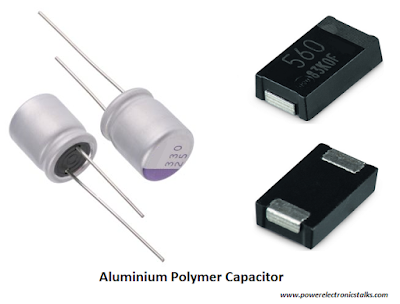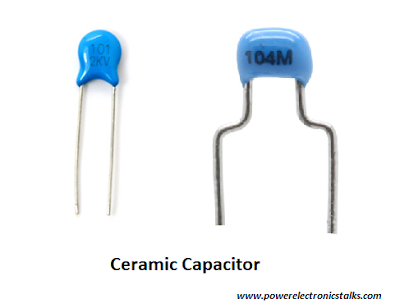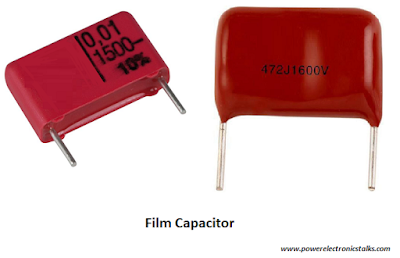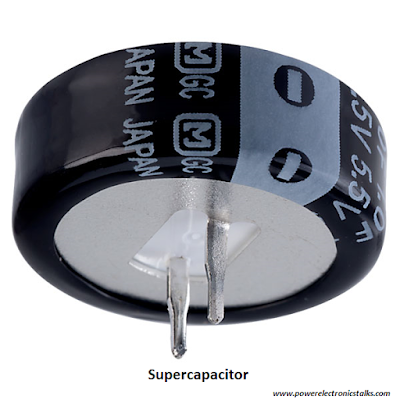# Capacitor Lifespan Calculations

We know that the operational conditions of a circuit directly affect the capacitor lifespan. The ambient temperature has the largest consequences on the lifespan of a capacitor. These consequences happen with all type of capacitors.

The relationship between capacitor lifespan and operating temperature follows Arrhenius' Law of Chemical Activity, which says that lifespan of a capacitor doubles for every 10°celsius decrease in the temperature.

## Capacitor Lifespan Calculations

Below are the formulas for capacitor lifespan calculations for different type of capacitors. These formulas represent the relationship between lifespan of a capacitor and it’s operating conditions.

#### 1) Radial, SMD and Snap-in electrolytic capacitor lifespan

We know that an aluminium electrolytic capacitor consists of an anode that have a thin aluminium foil with an etched surface. This surface is covered with an aluminium oxide which acts as a dielectric. Electrolytic capacitors offer largest capacitance value per unit volume as compare to other types of capacitors like ceramic capacitors and film capacitors.Radial, SMD, Snap-In Capacitor Lifespan
Below is the formula for calculations of lifespan of radial, SMD and snap-in electrolytic capacitors.

Le = Ll x (Vr/Va)ⁿ x 2ᴬ x 2ᴮ

Where;

Le = Expected lifespan of Radial, SMD and Snap-in electrolytic capacitor.

Ll = Load lifespan rating of the Radial, SMD and Snap-in electrolytic capacitor.

A = (Tₒ – Tₓ)/10

B = (ΔTₒ - ΔT)/C

Tₒ = Temperature rating of capacitor.

Tₓ = Ambient temperature of application.

ΔTₒ = 5 for 105°C capacitors

= 10 for 85°C capacitors

= 0 for the capacitors which are not rated with ripple current.

ΔT = C x (Ia/Ir)²

Ir = Rated ripple current of capacitor

Note: As per part specifications it may be increased by suitable frequency multiplier.

As temperature is already accounted for term “A” in the formula, don’t use temperature multiplier for this term.

Rated current i.e. the maximum current that may be applied, can be calculate as;

Datasheet value x Frequency multiplier x Temperature multiplier

But “lr” used in this equation is; Datasheet value x Frequency multiplier.

Ia = Applied ripple current (limited to catalogue specifications and its ripple current multipliers).

C = 5 for 105°C capacitors

= 10 for 85°C capacitors

Vr = Capacitor’s rated voltage

Va = Applied voltage

Vr/Va limited to a ratio of 2:1

n= 1 for Vr≥160V, n = 0 for Vr≤160V

#### 2) Axial electrolytic capacitor lifespan

For radial electrolytic capacitor both leads comes out of one side of the capacitor, but for an axial lead electrolytic capacitor has leads which come out at two opposite ends. Axial leaded electrolytic capacitors are perfect for applications having low profile circuit board.Axial Electrolytic Capacitor Lifespan
Below is the formula for calculations of lifespan of axial electrolytic capacitors.

Le = Ll x 2ˣ

Where;

Le = Expected lifespan of axial electrolytic capacitor in the application.

Ll = Load lifespan rating of axial electrolytic capacitor.

X = (Tm – Ta – ΔT)/10

ΔT = I² x ESR / (B x A)

Tm = Axial electrolytic capacitor’s maximum temperature rating.

Ta = Ambient temperature of the application.

ΔT = Temperature rise because of the ripple current.

I = Ripple current applied to the axial electrolytic capacitor.

ESR = Equivalent series resistance of axial electrolytic capacitor at application frequency.

B = Thermal constant.

A = Surface are of the axial electrolytic capacitor.

#### 3) Aluminium polymer capacitor lifespan

It consists of an aluminium anode, an aluminium cathode and a separator sheet which is infused with electrolyte. These capacitors have large capacitance along with low ESL and low ESR. Because of these characters, polymer capacitors are used in the applications like automotive applications and portable electronic equipment’s.Aluminium Polymer Capacitor Lifespan
Below is the formula for calculations of lifespan of aluminium polymer capacitors.

Le = Ll x 10ᶻ

Where;

Le = Expected lifespan of aluminium polymer capacitor.

Ll = Load lifespan rating of the aluminium polymer capacitor.

Z = (Tm – Ta)/20

Tm = Aluminium polymer capacitor’s maximum temperature rating.

Ta = Ambient temperature of the application.

4) Ceramic capacitor lifespan

Ceramic capacitors consists ceramic material that acts as the dielectric and are generally used mostly in all electronic applications. There’s no polarity.Ceramic Capacitor Lifespan
Below is the formula for calculations of lifespan of ceramic capacitors.

Le = Ll x (Vr/Va)³ x (Tm/Ta)⁸

Where;

Le = Expected lifespan of ceramic capacitor in the application.

Ll = Load lifespan rating of ceramic capacitor.

Tm = Ceramic capacitor’s maximum temperature rating.

Ta = Ambient temperature of the application.

Vr = Capacitor’s rated voltage.

Va = Applied voltage.

#### 5) Film capacitor lifespan

These capacitors are generally used in AC and DC electronic applications. Polypropylene film capacitor, plastic film capacitors and polymer film capacitors are most commonly used.Film Capacitor Lifespan
Below is the formula for calculations of lifespan of film capacitors.

Le = Ll x (Vr/Va)⁷ x 2ˣ

Where;

Le = Expected lifespan of film capacitor in the application.

Ll = Load lifespan rating of film capacitor.

X = (Tm – Ta – ΔT)/10

ΔT = I² x ESR / (B x A)

Tm = Film capacitor’s maximum temperature rating.

Ta = Ambient temperature of the application.

ΔT = Temperature rise because of the ripple current.

I = Ripple current applied to the film capacitor.

ESR = Equivalent series resistance of film capacitor at application frequency.

B = Thermal constant.

A = Surface are of the film capacitor.

Vr = Capacitor’s rated voltage.

Va = Applied voltage.

#### 6) Supercapacitor lifespan

Super capacitors have two layers of same material to store the electric charges, instead of a conventional dielectric component.Supercapacitor Lifespan
Below is the formula for calculations of lifespan of super capacitors.

Le = Ll x 2ˣ x 2ʸ

Where;

Le = Expected lifespan of supercapacitor in the application.

Ll = Load lifespan rating of the supercapacitor.

X = (Tm – Ta)/10

Y = (Vr – Va)/0.2

Tm = Supercapacitor’s maximum temperature rating.

Ta = Ambient temperature of the application.

Vr = Supercapacitor’s rated voltage.

Va = In the application, maximum applied voltage on the supercapacitor.

Below links of past articles from this blog will give you more details about capacitors;

"Capacitor working"

"Types of Capacitor"

"Uses of Capacitor"

### Conclusion

Calculation of capacitor lifespan will help designers to estimate product lifespan. These calculations will help them to select better capacitors for their designs.